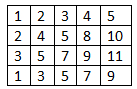# Find a common element in all rows of a given row-wise sorted matrix in C++

Suppose we have a matrix where each row is sorted. We have to write a function that will find the common elements in each row. Suppose the matrix is like below −The result will be 5.

To solve this, we will use hash based approach. This approach can also be used when the rows are not sorted. We have to follow some steps to do this −

We will create one hash table with all keys as distinct elements of two 1. All values will be 0

loop through each element in the matrix, if the number is present in the hash table then increase the count by 1. finally check whether if there is some value whose count is same as the row number of the matrix. If so then that is present in each row. (Assuming one value is not repeating in one row)

## Example

Live Demo

#include<iostream>
#include<unordered_map>
#define M 4
#define N 5
using namespace std;
int getCommonElement(int matrix[M][N]) {
unordered_map<int, int> count;
int i, j;
for (i = 0; i < M; i++) {
count[matrix[i]]++;
for (j = 1; j < N; j++) {
if (matrix[i][j] != matrix[i][j - 1])
count[matrix[i][j]]++;
}
}
for (auto ele : count) {
if (ele.second == M)
return ele.first;
}
return -1;
}
int main() {
int matrix[M][N] = {
{ 1, 2, 3, 4, 5 },
{ 2, 4, 5, 8, 10 },
{ 3, 5, 7, 9, 11 },
{ 1, 3, 5, 7, 9 },
};
int result = getCommonElement(matrix);
if (result == -1)
cout << "No common element has found";
else
cout << "Common element is " << result;
}

### Output

Common element is 5